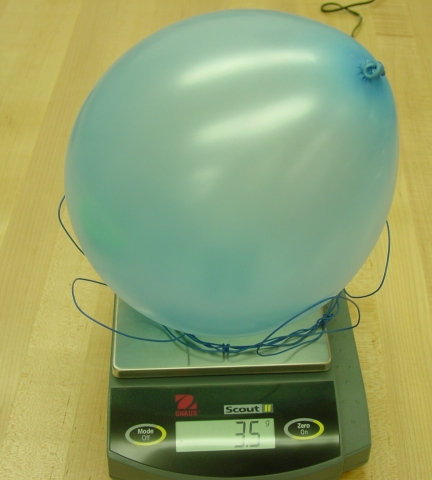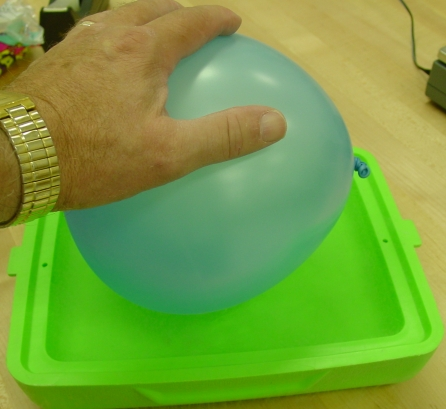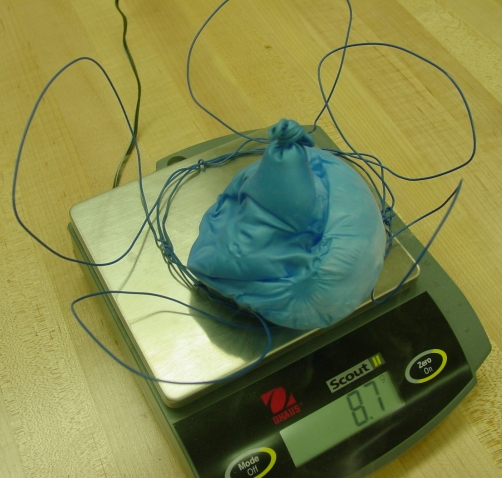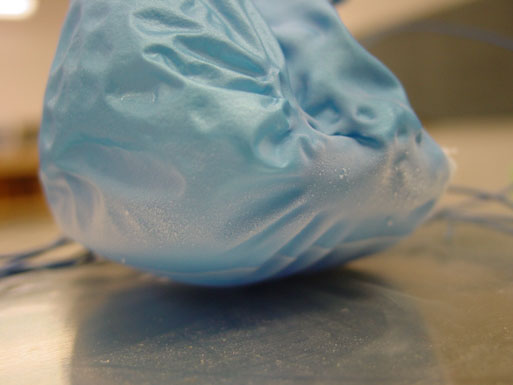# Experiment of The Month

## Upcoming Senior Seminar Projects

Wind makes it clear that air has mass, and with diligence, the density of air can be determined from the force by wind on a sail. A more transparent measurement of air density can be made by determining the weight of a sample of air.This demonstration is often done following a discussion of the ideal gas equation of state, PV=nRT. Some previous demonstrations show small effects of temperature on volume. The present demonstration is more dramatic.

We begin by weighing a balloon, then blowing it up and weighing it again. In the photo shown at right, the mass indication increased from 3.4 to 3.5 grams. At this point, it is important to note that the scale measures force, even though it reports a conclusion about mass based on the force measurement.

One assumption made in reaching the conclusion is that the buoyant force on the object being weighed is negligible. In the case of the balloon, this is incorrect. The buoyant force on this balloon is equal to the weight of the air displaced.

Since the volume of air inside the balloon is essentially the same as the volume of air displaced, we should expect that the buoyant force would support the weight of the air inside the balloon: The reported mass should not go up at all, because the force required of the scale should not change.

The increase in reported mass of .1 gram is attributed to higher density of air inside the balloon: The tension in the balloon compresses the air inside, as attested by the pressure required to blow the balloon up. Evidently for this experiment, the pressure inside is greater than atmospheric by about 2%.In the picture at right, the balloon is being pressed into a pan of liquid nitrogen. (The pan is the styrofoam lid of a small lunch box.) The balloon floats lightly on the liquid nitrogen unless pressed down. Pressing down places more surface area in contact with the cold nitrogen and speeds the demonstration. It is interesting to note the buoyant force by this liquified constituent of air.

The balloon shrinks dramatically, as indicated below. When left in contact with the liquid nitrogen long enough (perhaps 5 minutes) the oxygen inside the balloon liquifies, and then the nitrogen liquifies also. Close observation of the photo at the upper left corner of the pan shows some liquid nitrogen bubbles may forming above the dark spot in the center of the pan. One can also make out a faint line at the upper left corner of the pan which is the liquid nitrogen surface. The balloon still floats, riding rather high on that surface. Evidently, some of the balloon contents remain in the gas phase, making the mass of the balloon less than the mass of the displaced liquid nitrogen.Next, we take the shrunken balloon and place it back on the scale, as above. In this instance, the reported mass is 8.7 grams, an increase of 5.2 grams.

A look at the figure on the right shows a faint line near the bottom of the cold balloon. Above that line, the balloon contains gas; below liquid. That line represents the top surface of the liquid air inside the balloon. With this evidence, the easy thing to say would be, "Of course, liquids are heavier than gases," but that would be incorrect. We assert that the amount of air inside the balloon has not changed, and that the mass of that air is not dependent of temperature.

If these assertions are true, then the force of gravity on the balloon has not changed. The scale reading is determined by the force which it must exert on the balloon in order to keep it stationary. Evidently, the required force is larger when the balloon is shrunken. The reason is that the buoyant force (upward) has decreased to practically zero, leaving the scale alone to balance the downward force by gravity.

From the data, we can say that the change in the buoyant force is equal to the weight associated with the apparent change in mass. The weight of 5.2 grams is about .052 newtons. The buoyant force is less now because the balloon displaces less air. If we could measure the change in volume of the balloon as DV, then the buoyant force would be (r g DV) upwards, where r is the density of air that was displaced by the balloon, and g is the gravitational field strength, 9.8 Newton/kg.

Note that the .052 newton force is not the weight of the air inside the balloon. Rather, it is the weight of the air that was displaced by the balloon. If we ignore the compression of air inside the balloon, the two numbers are the same. However, the two samples of are completely different.

We can estimate the volume of the balloon by assuming that the hand in the photograph is about .1meters across. For purposes of estimation, we say that the volume shrank to almost zero when the balloon was cold, so that the change in volume was nearly equal to the original volume. Plugging in numbers gives fair agreement with the book value of 1kg/cubic meter for the density of air.

The value for the density of air is secondary to two main features of this demonstration:
1) Large changes in temperature produce the large changes in volume that are indicated by the ideal gas equation.
2) The mass of air in a volume equal to the volume of a balloon can be determined provided that the buoyant force is understood.

## Experiment Of The Month

View past labs experiments.